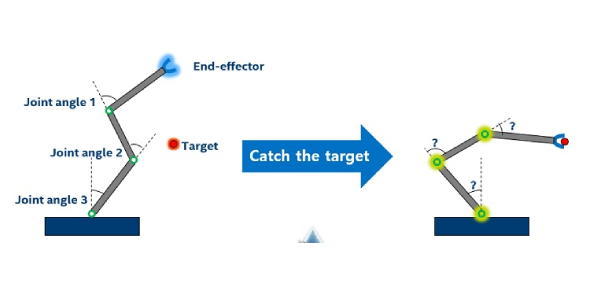# JEE Main Physics: Kinematics Quiz Set 1

10 Questions | Attempts: 325
ShareSettings.

• 1.
Going from city A to city B, a car accelerates uniformly from rest, then moves with constant velocity, and finally retards uniformly. If the ratio of time taken is 1:6:1 and the maximum speed is 80 km h-1, Then what is the average speed over the journey?
• A.
• B.
• C.
• D.
• 2.
A body is released from top of a tower of height X m. After 1 s, the body I stopped and then instantaneously released. What will be its height (from the ground) after next 2 s? (take g = 10 m/s2)
• A.

(X - 5)

• B.

(X - 25)

• C.

(X - 20)

• D.

(X - 40)

• 3.
A body travels 80 m in first 2s and 100 m in the next 4s with deceleration. What will be the velocity of the body at the end of 5th second?
• A.

5 m/s

• B.

25 m/s

• C.

20 m/s

• D.

40 m/s

• 4.
An object starts from rest and travels a distance 3D with uniform acceleration, then moves a distance 4D with uniform velocity and finally comes to rest after moving a distance of 5D under uniform retardation. What is the ratio of the average velocity to maximum velocity?
• A.

1/3

• B.

3/5

• C.

4/7

• D.

8/9

• 5.
An object is released from the top of a tower. Which of the following statement is correct about the time taken by the particle in falling through the successive distance of 1 m each?
• A.

All will be equal

• B.
• C.
• D.

None of These

• 6.
A passenger is standing on the platform. The passenger is standing at the beginning of the fourth coach of a train. How many coaches are there on the train if it takes 6 seconds by the fourth coach and 24 seconds by the rest of the train (including 4th coach) to cross the passenger, then how many coaches are there in the train?
• A.

13

• B.

18

• C.

15

• D.

17

• 7.
An object starts sliding from the top of a triangular box with a base of 15 inches and a height of 8 inches. The Inclined height of the box is divided into three parts in such a way that the object takes equal times to slide down these parts. What will be the length of these parts?
• A.

15/9, 5, 75/9

• B.

20/9, 60/9, 100/9

• C.

17/9, 51/9, 85/9

• D.

10/9, 30/9, 50/9

• 8.
A ball is released from the top of a tower. Another ball is released from a point ‘H2’ meter below the top when the first ball has descended ‘H1’ meter from the top. If booth ball are released from rest and reach the ground simultaneously then what is the height of the tower?
• A.
• B.
• C.
• D.
• 9.
A heavy stone is dropped into a well in which the water level is at depth H below the ground level. If the speed of the sound is ‘v’ then the time after which the splash is heard will be given by
• A.
• B.
• C.
• D.
• 10.
The height of a container is 1.5 m is being lifted up by a crane, with an acceleration of 2 m/s2. One second after the start, a bolt from the roof of the container starts falling. What will be the displacement of the bolt before touching the floor of the container?
• A.

3/5

• B.

3/8

• C.

2/3

• D.

1/4

## Related TopicsBack to top
×

Wait!
Here's an interesting quiz for you.